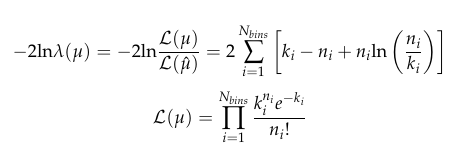# NLL and Poisson pdf

Dear Root Forum user,

I am trying to extract my signal strength minimizing the function:where k_{i}= \mu \times s_{i} + b_{i}, being \mu the fit parameter and the other the signal/background expected number of events that I have. The  n_{i} is the number of observed events following a Poisson distribution.
From what I saw, the RooFit seemed to me to be the best option to perform this fit. I already make some tests to generate a set of n_{i} using a Poisson distribution. However, in my way, for a large range, the code will take a long time to get an output, and it is inefficient.

    vector<int>Vect={1,2,3,4,5,6,7,8,9,10};
RooRealVar l("X","X",0,20);
RooRealVar mean("mean","mean",0,10);
//RooDataHist hl("hist","hist",RooArgSet(mean,p));
RooDataSet dl("set","set",RooArgSet(mean));

for(Int_t i=1; i<11; i++) // loop para criar um Set das médias
{
mean = i;

}
vector<double_t>Vect2;
RooDataSet plx("X","X",RooArgSet(l));

for (Int_t i = 0; i < dl.sumEntries(); i++)
{
TCanvas *c1 = new TCanvas("c1","sd",900,900);
RooRealVar p("p","p",0,30);
const RooArgSet &argSet = *(dl.get(i));
argSet.Print("V");
double_t var = static_cast<RooAbsReal&>(argSet["mean"]).getVal();
//cout << "var: " << var << endl;
RooRealVar X("X","X",var,0,20);
RooPoisson* Ppoisson = new RooPoisson("Ppoisson","poisson with n",p,X);

RooDataSet *dataV = Ppoisson->generate(p,1000);
Ppoisson->fitTo(*dataV);
cout << "*****************" << endl;
cout <<" X is";
X.Print();
cout << endl;
//cout << "---------------------------" << X.get()<< "------------------"<<endl;
RooPlot* xframe = p.frame();
dataV->plotOn(xframe);
Ppoisson->plotOn(xframe);
xframe->Draw();
c1->Print(Form("PoisonB_p%i.png",Vect.at(i)));
}

for (Int_t i = 0; i < plx.sumEntries(); i++)
{
cout << "plx"<< endl;
plx.get(i)->Print("V");
cout << "............"<< endl;

}


What is the best way to follow to resolve this? RooFit is the best option for find n_{i} and for the NLL?

Thanks a lot,

Francisco

Hi @FCasa23; maybe @jonas can help here with RooFit.

Cheers,
J.

This topic was automatically closed 14 days after the last reply. New replies are no longer allowed.